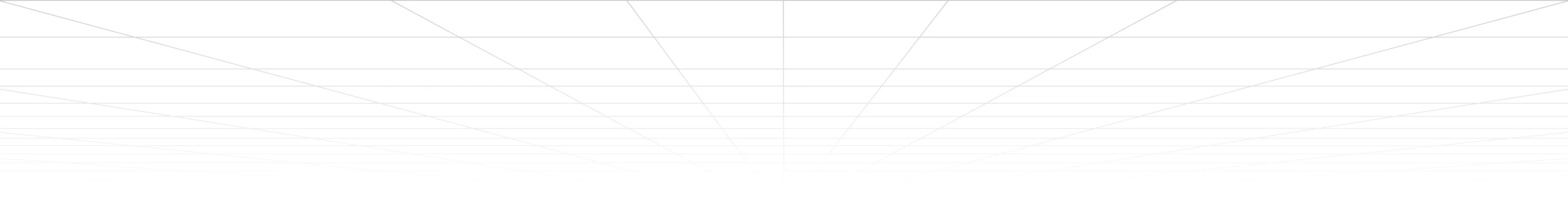Introducing WorkLMTM
Leena AI's Proprietary Large Language Model That Makes Work EasyWhat's new
What are Markov Models?
Markov models, also known as Markov processes or Markov chains, are probabilistic models that capture the dynamics and dependencies of a sequence of events. Markov models are widely used to model and generate sequences of data, such as text, music, or time series.
At the core of a Markov model is the Markov property, which states that the future state of a system depends only on its current state and is independent of its past states. This property allows the model to simplify complex sequences by assuming that the probability distribution of the next event depends only on the current event.In a Markov model, a sequence of events is represented as a series of states, and the transitions between states are governed by probabilities. The model can be represented as a directed graph, where each state is a node, and the probabilities of transitioning between states are represented by the edges.
The order of a Markov model refers to the number of previous states considered when predicting the next state. For example, in a first-order Markov model (also known as a Markov chain), the next state depends only on the current state. In a second-order Markov model, the next state depends on the current state as well as the previous state.
Training a Markov model involves estimating the transition probabilities from a given dataset. Given a sequence of events, the model calculates the probabilities of transitioning from one state to another based on the observed frequencies in the data. The training process aims to capture the statistical patterns and dependencies present in the dataset.
Back to glossary